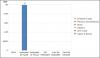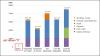Empty <set/> in stacked 2d throwing "Y is undefined" js error

Recommended Posts

Hi, can we bulild empty set tag in stacked 2d Chart

When im trying to build <set value=""> or <set value=null> or <set/>,Iam getting Js Exception.

I need to build chart as shown in "Requirment.jpg"

When im setting value="0" ShowSum is displaying sum on Top as 0.

Can someone please tell me how to build chart with empty set tag Its important and immediate requirment.Share on other sites

My Xml is as follwos.

<categories>
<category name="GDFV"/>
<category name="Realizable at Target"/>
<category name="Realizable at Payout"/>
<category name="ISS Realizable"/>
<category name="Conf Bd Realizable"/>
<category name="Conf Bd Realized"/>

</categories>

<dataset seriesName="Salary">
<set value="420"/>
<set value="295"/>
<set value="523"/>
<set value="473"/>
<set/>
<set/>

<set value="354"/>
<set value="457"/>
<set value="127"/>
<set value="354"/>
<set value="485"/>
<set value="486"/>
</dataset>

<dataset seriesName="Bonus">
<set value="420"/>
<set value="295"/>
<set value="523"/>
<set value="473"/>
<set/>
<set/>

<set value="354"/>
<set value="457"/>
<set value="127"/>
<set value="354"/>
<set value="485"/>
<set value="486"/>
</dataset>

<dataset seriesName="LtipCash">
<set value="420"/>
<set value="295"/>
<set value="523"/>
<set value="473"/>
<set/>
<set/>

<set value="354"/>
<set value="457"/>
<set value="127"/>
<set value="354"/>
<set value="485"/>
<set value="486"/>
</dataset>

ERROR:

TypeError: y is undefined

Share on other sites

Hi,

Do check with our latest release and let us know if your issue has been resolved?

Share on other sites

Could you be more specific in version, where this issue is fixed??

Share on other sites

I have verified in my application, we are using Fusioncharts version 3.5.1. But still we are facing this issue. Please advice?